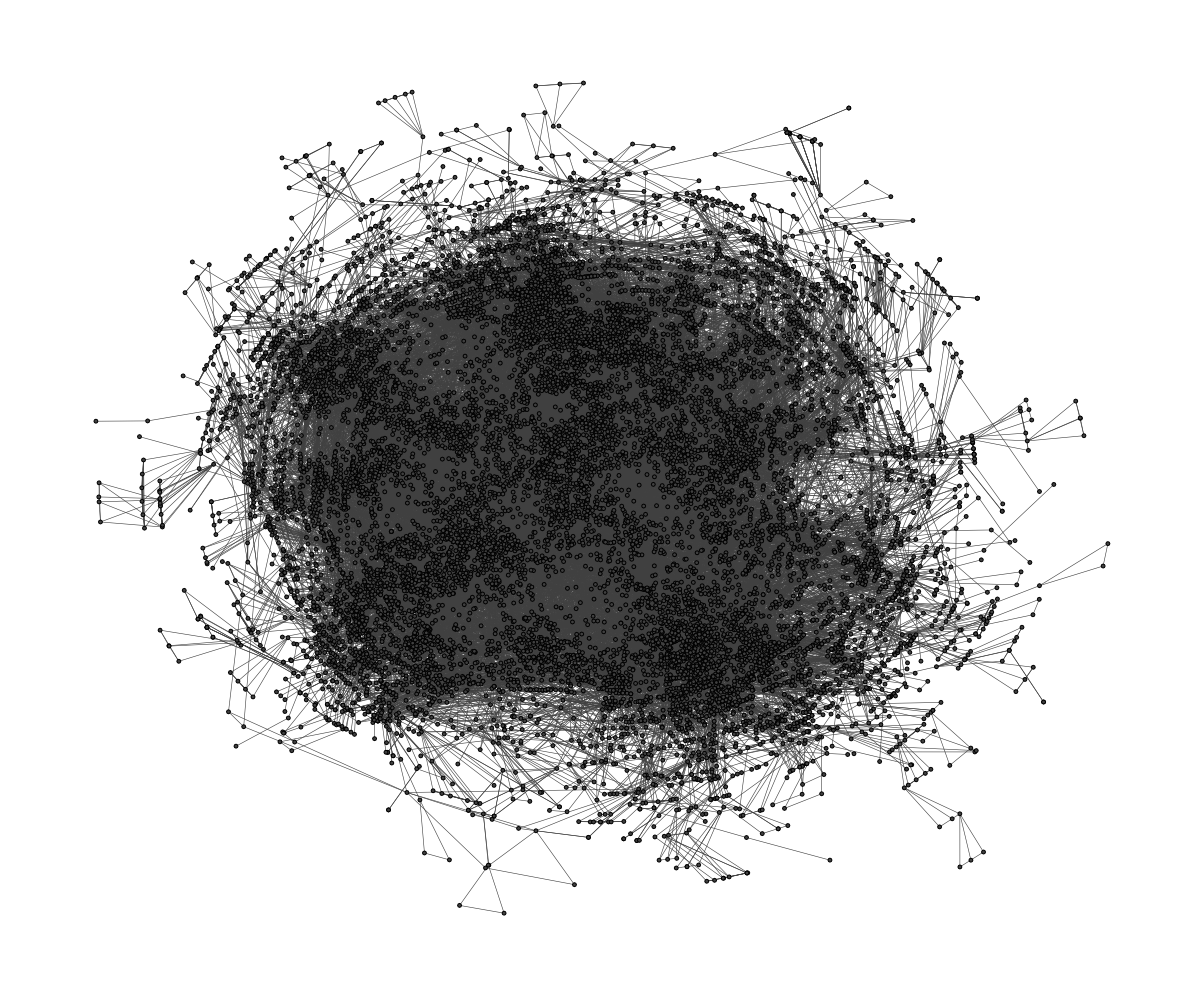# graphlayouts

This package implements some graph layout algorithms that are not available in `igraph`.

A detailed introductory tutorial for graphlayouts and ggraph can be found here. (version 0.2.0/ggraph 1.0.2)

So far, the package implements the following algorithms:

• Stress majorization (Paper)
• sparse stress (Paper)
• pivot MDS (Paper)
• dynamic layout for longitudinal data (Paper)
• spectral layouts

## Install

``````# dev version
remotes::install_github("schochastics/graphlayouts")

#CRAN
install.packages("graphlayouts")``````

## Stress Majorization: Connected Network

This example is a bit of a special case since it exploits some weird issues in igraph.

``````library(igraph)
library(ggraph)
library(graphlayouts)

set.seed(666)
pa <- sample_pa(1000,1,1,directed = F)

ggraph(pa,layout = "nicely")+
geom_node_point(col="black",size=0.3)+
theme_graph()````````````
ggraph(pa,layout="stress")+
geom_node_point(col="black",size=0.3)+
theme_graph()``````## Stress Majorization: Unconnected Network

Stress majorization also works for networks with several components. It relies on a bin packing algorithm to efficiently put the components in a rectangle, rather than a circle.

``````set.seed(666)
g <- disjoint_union(
sample_pa(10,directed = F),
sample_pa(20,directed = F),
sample_pa(30,directed = F),
sample_pa(40,directed = F),
sample_pa(50,directed = F),
sample_pa(60,directed = F),
sample_pa(80,directed = F)
)

ggraph(g,layout = "nicely") +
geom_node_point() +
theme_graph()````````````
ggraph(g, layout = "stress",bbox = 40) +
geom_node_point() +
theme_graph()``````## Backbone Layout

Backbone layouts are helpful for drawing hairballs.

``````set.seed(665)
#create network with a group structure
g <- sample_islands(9,40,0.4,15)
g <- simplify(g)
V(g)\$grp <- as.character(rep(1:9,each=40))

ggraph(g,layout = "stress")+
geom_node_point(aes(col=grp))+
scale_color_brewer(palette = "Set1")+
theme_graph()+
theme(legend.position = "none")``````The backbone layout helps to uncover potential group structures based on edge embeddedness and puts more emphasis on this structure in the layout.

``````bb <- layout_as_backbone(g,keep=0.4)
E(g)\$col <- F
E(g)\$col[bb\$backbone] <- T

ggraph(g,layout="manual",x=bb\$xy[,1],y=bb\$xy[,2])+
geom_node_point(aes(col=grp))+
scale_color_brewer(palette = "Set1")+
scale_edge_color_manual(values=c(rgb(0,0,0,0.3),rgb(0,0,0,1)))+
theme_graph()+
theme(legend.position = "none")``````## Radial Layout with Focal Node

The function `layout_with_focus()` creates a radial layout around a focal node. All nodes with the same distance from the focal node are on the same circle.

``````library(igraphdata)
library(patchwork)
data("karate")

p1 <- ggraph(karate,layout = "focus",focus = 1) +
draw_circle(use = "focus",max.circle = 3)+
geom_node_point(aes(fill=as.factor(Faction)),size=2,shape=21)+
theme_graph()+
theme(legend.position = "none")+
coord_fixed()+
labs(title= "Focus on Mr. Hi")

p2 <- ggraph(karate,layout = "focus",focus = 34) +
draw_circle(use = "focus",max.circle = 4)+
geom_node_point(aes(fill=as.factor(Faction)),size=2,shape=21)+
theme_graph()+
theme(legend.position = "none")+
coord_fixed()+
labs(title= "Focus on John A.")

p1+p2``````The function `layout_with_centrality` creates a radial layout around the node with the highest centrality value. The further outside a node is, the more peripheral it is.

``````library(igraphdata)
library(patchwork)
data("karate")

bc <- betweenness(karate)
p1 <- ggraph(karate,layout = "centrality", centrality = bc, tseq = seq(0,1,0.15)) +
draw_circle(use = "cent") +
annotate_circle(bc,format="",pos="bottom") +
geom_node_point(aes(fill=as.factor(Faction)),size=2,shape=21)+
theme_graph()+
theme(legend.position = "none")+
coord_fixed()+
labs(title="betweenness centrality")

cc <- closeness(karate)
p2 <- ggraph(karate,layout = "centrality", centrality = cc, tseq = seq(0,1,0.2)) +
draw_circle(use = "cent") +
annotate_circle(cc,format="scientific",pos="bottom") +
geom_node_point(aes(fill=as.factor(Faction)),size=2,shape=21)+
theme_graph()+
theme(legend.position = "none")+
coord_fixed()+
labs(title="closeness centrality")

p1+p2``````## Large graphs

`graphlayouts` implements two algorithms for visualizing large networks (<100k nodes). `layout_with_pmds()` is similar to `layout_with_mds()` but performs the multidimensional scaling only with a small number of pivot nodes. Usually, 50-100 are enough to obtain similar results to the full MDS.

`layout_with_sparse_stress()` performs stress majorization only with a small number of pivots (~50-100). The runtime performance is inferior to pivotMDS but the quality is far superior.

A comparison of runtimes and layout quality can be found in the wiki
tl;dr: both layout algorithms appear to be faster than the fastest igraph algorithm `layout_with_drl()`.

Below are two examples of layouts generated for large graphs using `layout_with_sparse_stress()`A retweet network with ~18k nodes and ~61k edges (runtime: 45.2s)A co-citation network with ~12k nodes and ~68k edges (runtime: 21s)

## dynamic layouts

`layout_as_dynamic()` allows you to visualize snapshots of longitudinal network data. Nodes are anchored with a reference layout and only moved slightly in each wave depending on deleted/added edges. In this way, it is easy to track down specific nodes throughout time. Use `patchwork` to put the individual plots next to each other.

``````library(patchwork)
#gList is a list of longitudinal networks.

xy <- layout_as_dynamic(gList,alpha = 0.2)
pList <- vector("list",length(gList))

for(i in 1:length(gList)){
pList[[i]] <- ggraph(gList[[i]],layout="manual",x=xy[[i]][,1],y=xy[[i]][,2])+
geom_node_point(shape=21,aes(fill=smoking),size=3)+
geom_node_text(aes(label=1:50),repel = T)+
scale_fill_manual(values=c("forestgreen","grey25","firebrick"),guide=ifelse(i!=2,FALSE,"legend"))+
theme_graph()+
theme(legend.position="bottom")+
labs(title=paste0("Wave ",i))
}
Reduce("+",pList)+
plot_annotation(title="Friendship network",theme = theme(title = element_text(family="Arial Narrow",face = "bold",size=16)))``````## Layout manipulation

The functions `layout_mirror()` and `layout_rotate()` can be used to manipulate an existing layout